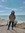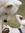# Philosophy discussion

91 views
Science and Mathematics > Nested Propositions : True False and Meaningless

message 1: by (new)According to Bertrand Russell (The Open Society and its Enemies: Vol II, Popper, K. ; 1945) there are three types of propositions, namely True, False and Meaningless or pseudo proposition. Now Popper (1945), if I understood him correctly, was against the idea of equating true proposition with proposition of natural science (Wittgenstein’s idea) as such a step would remove false hypothesis from the realm of science. And since we cannot know if a hypothesis is true or not, by definition, it becomes part of metaphysics and as such meaningless. Now let us take popper’s side and assume that there is a class of propositions (Y) which state that certain proposition, X, cannot be tested (i.e. proven or disproven). Now if (Y) is true then falsification theory cannot be applied to X but one can very well apply it to Y itself. Then Y becomes a testable hypothesis, which can be true false or meaningless.
Now we can construct a thought experiment, whereby we can state under what condition we can state Y is true or false. And so we can state if the result is Z1, then Y is true and if it is ‘not Z1’ then Y is false. Based on falsification paradigm, we should be looking for ‘not Z1’ and until we find it we can say Y is provisionally true. If the result is ‘not Z1’ then Y is false and X is indeed testable. If the result is Z1 then Y is provisionally true and X cannot be tested. But this in turn means X cannot be proven or disproven and therefore cannot be true or false and therefore is meaningless. Now what characteristic must ‘not Z1’ have is the important question, since upon it depend all outcomes; A proposition can be false in many ways and therefore Not Z1 includes all possible outcome other than Z1. Now ‘Not Z1’ implies X is testable; therefore if we can construct a hypothetical situation where we can state X is true or false then we have already proven Y is false (which is obvious). It seems the truth value of Y cannot be determined without trying to determine the truth value of X. If is either true or false then Y is false but if X is meaningless then Y is true. Thus if we fail to construct such a situation whereby we can state under this condition we can test X then Y is provisionally true and X is indeed meaningless.
Now to the question, let us take the hypothetical proposition “the statement ‘there is a God’ cannot be tested’, where ‘there is a God’ is X. Create a thought experiment which can prove or disprove X or simply take the situation (to help you visualize): Under what condition would a theist believe there is no God or vice versa an Atheist would believe there is a God. I could not find any condition which could satisfy or answer this question. Therefore X is meaningless and Y is true. And since Y is true it is part of the totality of true propositions (Wittgenstein) while X is not. The result does seem a bit trivial, much ado about nothing.

message 2: by (new)There are many examples of theists losing their faith. There are also lots of instances of athiests becoming religious. Thus, there are certainly conditions under which at least some theists come to believe that there is no God, and conditions where at least some theists believe there is a God. I find it hard to believe that any individual's inability to find such conditions is enough to establish the conclusion.

What about the following proposition: "A proposition which cannot be tested is meaningless." What test do you propose which could falsify this? I suggest this might be impossible. If that's so, then the Popper position, if true, is also meaningless.